# Mathematics 1010 online

## Never Divide by Zero

Division by zero is undefined, and for good reason. If we assigned a number to the result of dividing by zero we'd run into contradictions, and mathematics would become useless.

Let's approach the issue from several angles:

• Definition. Division is the inverse process of multiplication. For example, the unique (one and only) solution offor, is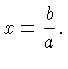When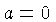we get the equationThis equation has no solution if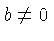, and any number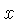will be a solution if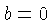. In neither case do we have a unique solution. This argument only shows that our usual way of defining division fails. The next argument shows that there is no way of extending the definition in a way that does not give rise to contradictions.

• A Contradiction. Suppose we define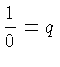for some real number. Multiplying on both sides of the equation gives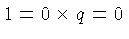which is a contradiction (toand 0 being different numbers).

• A more subtle contradiction. Try to figure out what's wrong with the following "proof" that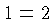, before reading the answer at the bottom of this page.

Let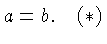Then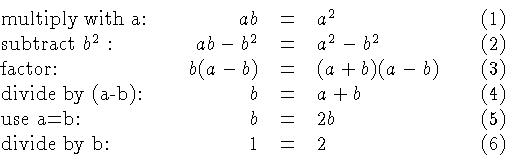• Another Fallacy. Temptations to divide by zero arise in subtle ways. For example, consider the equation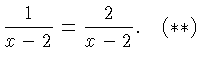The routine approach to solving this equation begins by taking reciprocals on both sides, giving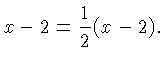Multiplying with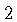on both sides givesFinally, adding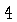and subtractingon both sides gives what might appear to be a "solution":.

However, when we setin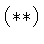we divide by zero on both sides of the equation and obtain nonsense. The equationhas no solution!

Of course, you would have discovered that fact by following another one of our basic principles:

In going from (3) to (4) we divide by zero sinceimplies that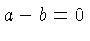.